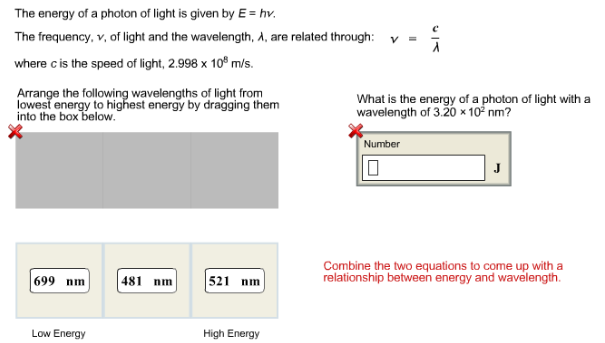# Problem: The energy of a photon of light is given by E = hv. The frequency, v, of light and the wavelength, λ, are related through: v = c/λ where c is the speed of light, 2.998 x 108 m/s. Arrange the following wavelengths of light from lowest energy to highest energy by dragging them into the box below. What is the energy of a photon of light with a wavelength of 3.20 x 102 nm?

###### FREE Expert Solution
79% (242 ratings)###### Problem Details

The energy of a photon of light is given by E = hv. The frequency, v, of light and the wavelength, λ, are related through: v = c/λ where c is the speed of light, 2.998 x 108 m/s. Arrange the following wavelengths of light from lowest energy to highest energy by dragging them into the box below.

What is the energy of a photon of light with a wavelength of 3.20 x 102 nm?